Homework Help Question & Answers

# 3.43 Find the formula for the n-th power of this matrix.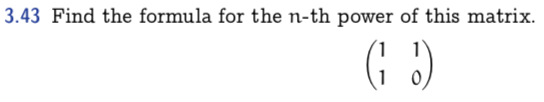3.43 Find the formula for the n-th power of this matrix.

#### Homework Answers

Answer #1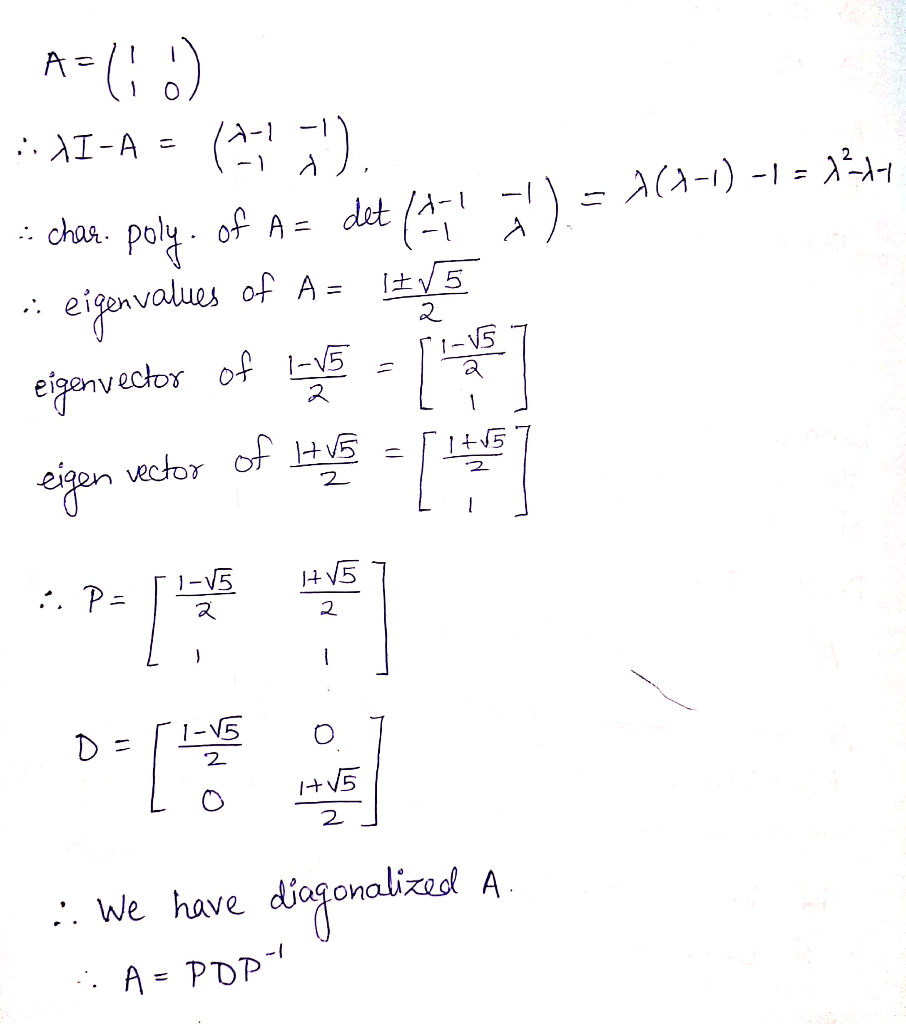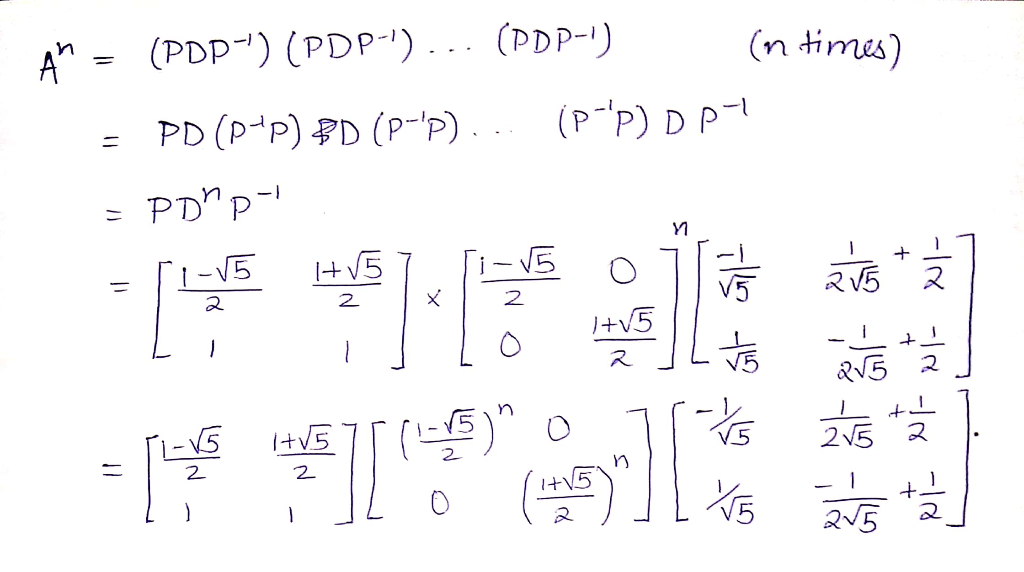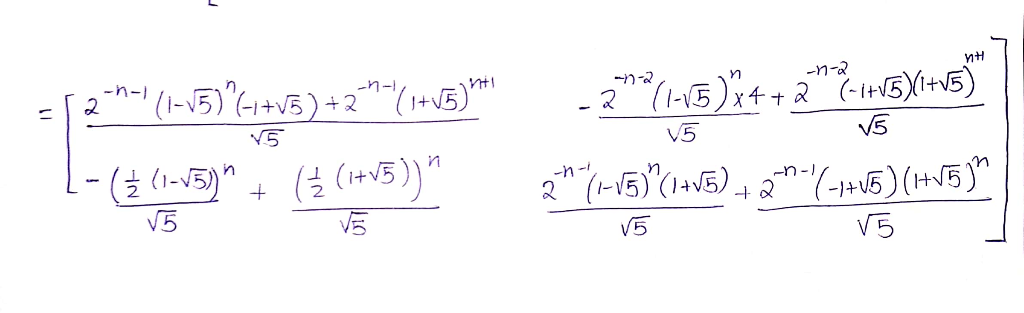Please upvote the answer

Know the answer?
Your Answer:

#### Post as a guest

Your Name:

What's your source?

#### Earn Coin

Coins can be redeemed for fabulous gifts.

Not the answer you're looking for? Ask your own homework help question. Our experts will answer your question WITHIN MINUTES for Free.
Similar Homework Help Questions
• ### k 5. Find the matrix power (3 :) for k € N.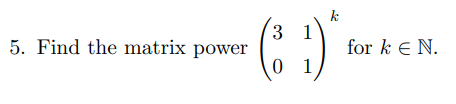k 5. Find the matrix power (3 :) for k € N.

• ### Write an algorithm that estimates the desired eigenvalues of an input matrix by using the power...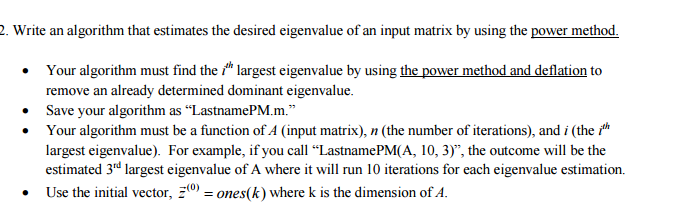Write an algorithm that estimates the desired eigenvalues of an input matrix by using the power method. Your algorithm must find the i^th largest eigenvalues by using the power method and deflation to remove an already determined dominant eigenvalues. Save your algorithm as "LastnamePM.m." Your algorithm must be a function of A (input matrix), n (the number of iterations), and i (the i^th largest eigenvalues). For example, if you call "LastnamePM(A, 10,3)", the outcome will be the estimated 3,d largest...

• ### Find the power of A for the matrix A = -1 0 0 0 - 1...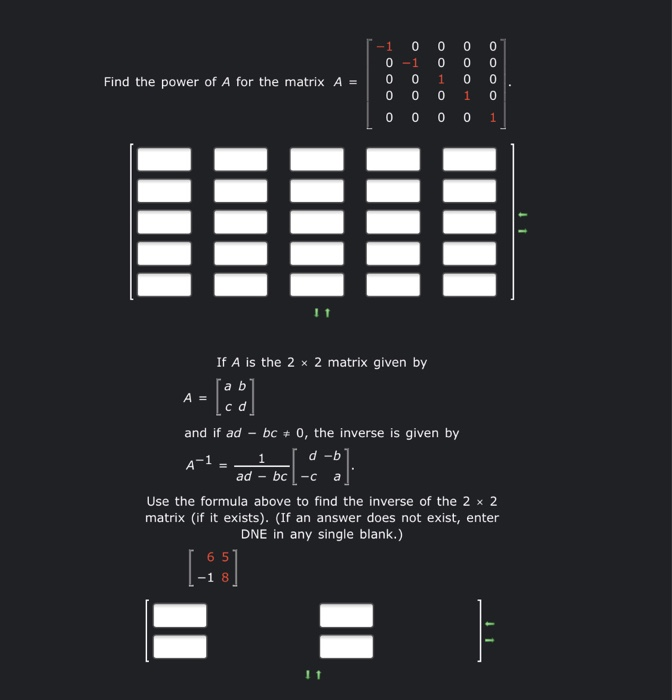Find the power of A for the matrix A = -1 0 0 0 - 1 0 0 0 0 OOOO OOOO 0 0 0 0 0 0 0 0 1 If A is the 2 x 2 matrix given by [aь A = cd and if ad - bc + 0, the inverse is given by d-b ad - bc Use the formula above to find the inverse of the 2 x 2 matrix (if it exists). (If an...

• ### (1 point) Find a function of x that is equal to the power series En= n(n...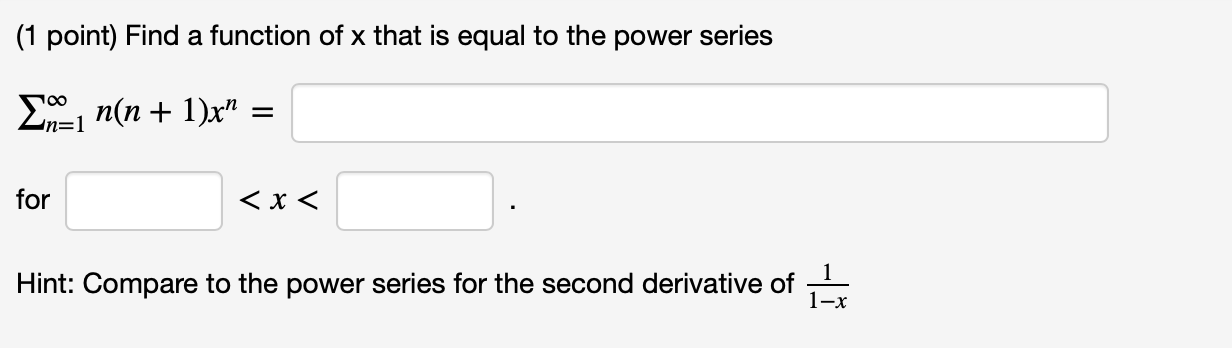(1 point) Find a function of x that is equal to the power series En= n(n + 1)x" = for <x< Hint: Compare to the power series for the second derivative of 1-X (1 point) Find a formula for the sum of the series (n + 1)x" n=0 101+2 for –10 < x < 10. Hint: D,( *) = " " 10n+1

• ### Suppose A is an n by n matrix that is diagonalized by P. Find a matrix that diagonalizes A^T

Suppose A is an n by n matrix that is diagonalized by P. Find a matrix that diagonalizes AT

• ### Let A be a diagonalizable n × n matrix and let P be an invertible n...

Let A be a diagonalizable n × n matrix and let P be an invertible n × n matrix such that B = P−1AP is the diagonal form of A. Prove that Ak = PBkP−1, where k is a positive integer. Use the result above to find the indicated power of A. A = −4 0 4 −3 −1 4 −6 0 6 , A5

• ### Let A be a diagonalizable n x n matrix and let P be an invertible n...Let A be a diagonalizable n x n matrix and let P be an invertible n x n matrix such that B = p-1AP is the diagonal form of A. Prove that A* = Pokp-1, where k is a positive integer. Use the result above to find the indicated power of A. 10 18 A = -6 -11 18].46 A = 11

• ### 2. Discrete Fourier Transform.(/25) 1. N-th roots of unity are defined as solutions to the equation:...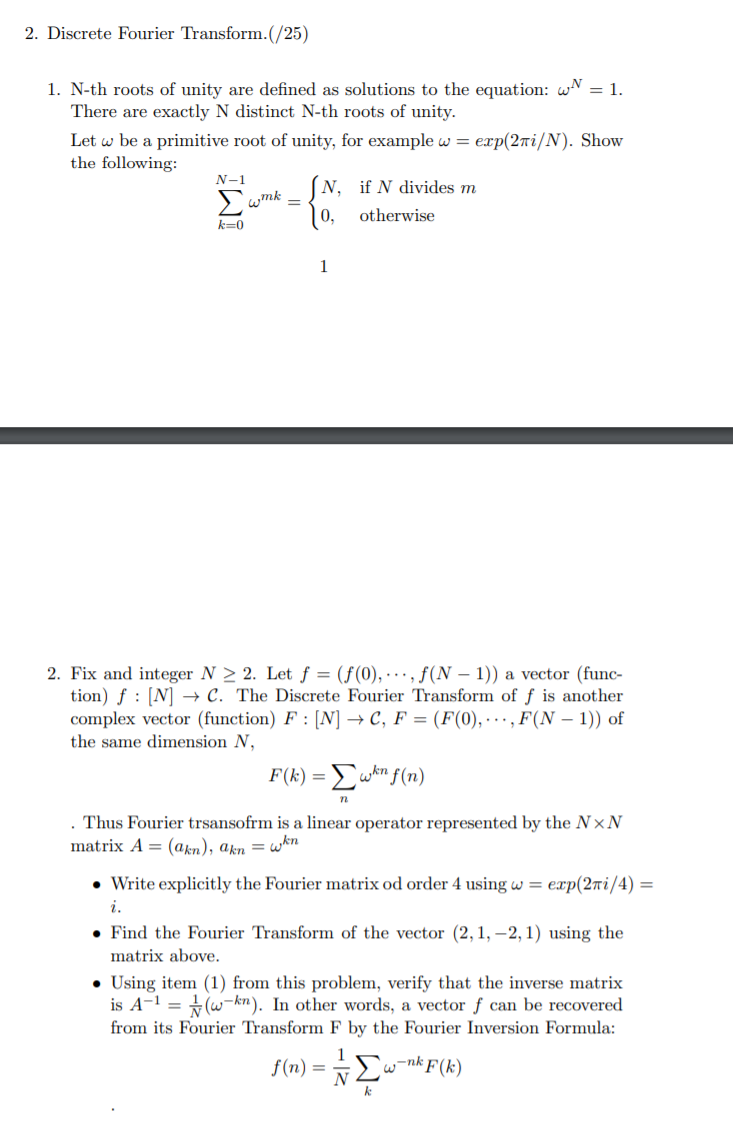2. Discrete Fourier Transform.(/25) 1. N-th roots of unity are defined as solutions to the equation: w = 1. There are exactly N distinct N-th roots of unity. Let w be a primitive root of unity, for example w = exp(2 i/N). Show the following: N, if N divides m k=0 10, otherwise N -1 N wmk 2. Fix and integer N > 2. Let f = (f(0), ..., f(N − 1)) a vector (func- tion) f : [N] →...

• ### Let A be a diagonalizable n x n matrix and let P be an invertible n...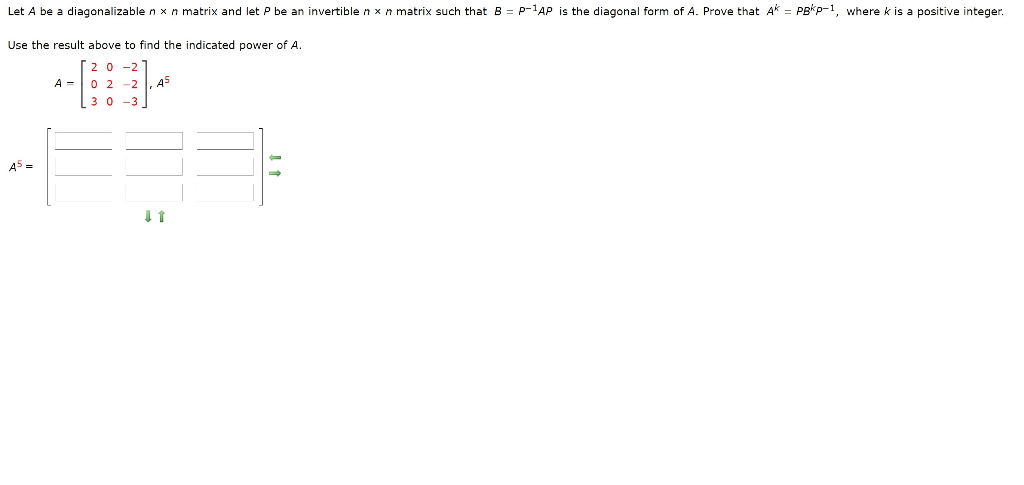Let A be a diagonalizable n x n matrix and let P be an invertible n x n matrix such that B = P-1AP is the diagonal form of A. Prove that Ak = Pekp-1, where k is a positive integer. Use the result above to find the indicated power of A. 0-2 02-2 3 0 -3 ,45 A5 = 11

• ### Find the characteristic polynomial of the matrix, using either a cofactor expansion or th 4 0...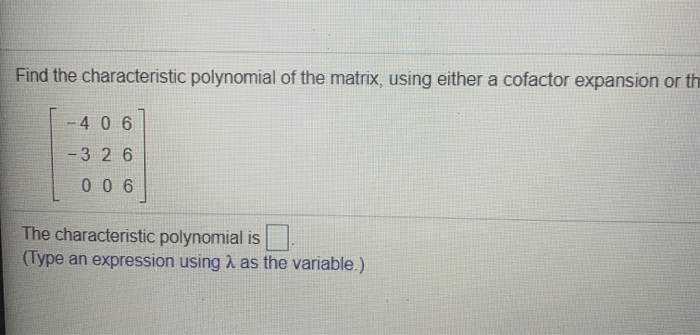Find the characteristic polynomial of the matrix, using either a cofactor expansion or th 4 0 6 - 3 2 6 006 The characteristic polynomial is (Type an expression using 2 as the variable.)

Free Homework App

Scan Your Homework
to Get Instant Free Answers
Need Online Homework Help?

Get Answers For Free
Most questions answered within 3 hours.